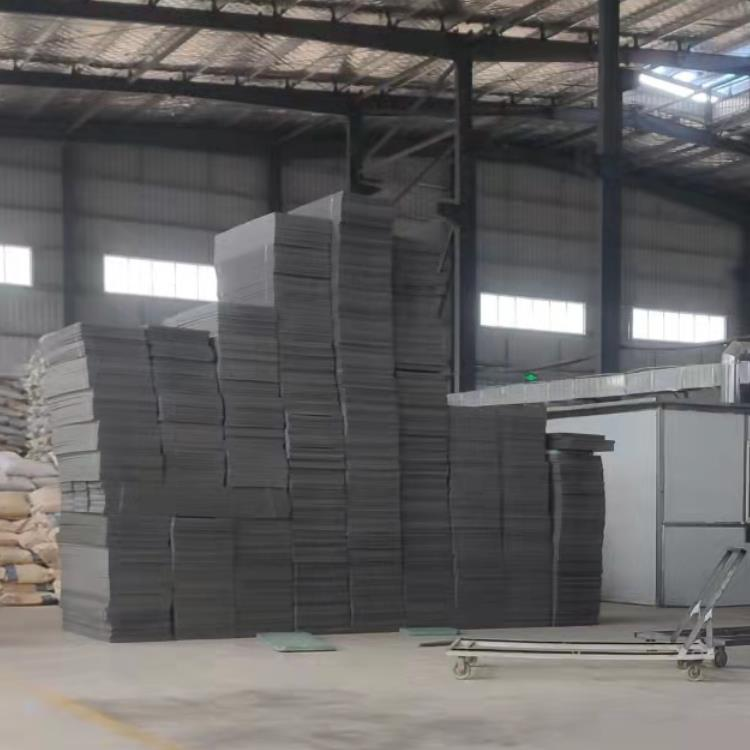" />

【 微信扫码咨询 】

13698775878

# 挤塑板性能参数带数据U=1/(1/10+0.15/1+1/30)=3.53W/m2K

q=3.53[W/m2K]x30[K]=105.9W/m2

q损失=q。A=105.9[W/m2]x30[m2]=3177W

U=1/(1/10+0.15/1+0.1/0.028+1/30)=0.259W/m2K

q=0.259[W/m2K]x30[K]=7.78W/m2

q损失=q。A=7.78[W/m2]x30[m2]=233W